# LINQ Except Method in C#

## LINQ Except Method in C# with Examples

In this article, I will discuss the LINQ Except Method in C# with Examples. Please read our previous article discussing the LINQ Distinct Method in C# with Examples. As part of this article, we will discuss the following pointers.

1. What is LINQ Except Method in C#?
2. Example to Understand Except Method with Value Types in C#
3. Example to Understand LINQ Except Method with String Data Type
4. What happens if any sequences are null while performing the Except Operation?
5. Example to Understand LINQ Except Method with String Data Type
6. Using Anonymous Type with Except Method in C#
7. Using IEqualityComparer Comparer with LINQ Except Method in C#
8. Overriding Equals() and GetHashCode() Methods
9. Implementing IEquatble<T> Interface
10. When to Use LINQ Except Method in C#?
##### What is LINQ Except Method in C#?

The LINQ Except Method in C# performs set difference operations between two collections. It returns elements from the first collection that are absent in the second. Two overloaded versions are available for the LINQ Except Method in C#. They are as follows.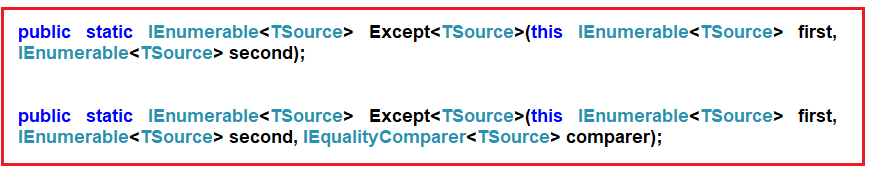The one and only difference between the above two methods is that the second overloaded version takes IEqualityComparer as an argument. That means the Except Method can also be used with Comparer. Let us understand the LINQ Except Method with an example. Please have a look at the following image.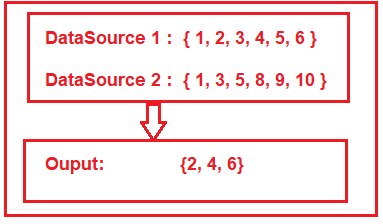As you can see in the above image, we have two data sources, i.e., DataSource 1 and Data Source 2. DataSource 1 contains elements 1, 2, 3, 4, 5, and 6, and DataSource 2 contains elements 1, 3, 5, 8, 9, and 10. If we want to retrieve the elements such as 2, 4, and 6 from the first data source, which do not exist in the second data source, then we need to apply the Except operation between DataSource 1 and Data Source 2. Let us see how to do this with both Query and Method Syntax using Except Method.

##### Example to Understand Except Method with Value Types in C#:

In the example below, I show how to use LINQ Except Method with Value Types using both Method and Query Syntax. In query syntax, there is no such operator call Except, so here, we have to use both query and method syntax to achieve the same.

```using System;
using System.Collections.Generic;
using System.Linq;
namespace LINQDemo
{
class Program
{
static void Main(string[] args)
{
List<int> dataSource1 = new List<int>() { 1, 2, 3, 4, 5, 6 };
List<int> dataSource2 = new List<int>() { 1, 3, 5, 8, 9, 10 };

//Method Syntax
var MS = dataSource1.Except(dataSource2).ToList();

//Query Syntax
var QS = (from num in dataSource1
select num)
.Except(dataSource2).ToList();

foreach (var item in QS)
{
Console.Write(item + " ");
}

}
}
}```

Run the application, and you will see the output as expected, i.e., 2 4 6.

##### What Happens if any of the Sequences is Null?

The Except Method will throw an exception if any sequences are null. In the example below, the second sequence is null, and performing the Except operation using the Except method will throw an exception.

```using System;
using System.Collections.Generic;
using System.Linq;
namespace LINQDemo
{
class Program
{
static void Main(string[] args)
{
List<int> dataSource1 = new List<int>() { 1, 2, 3, 4, 5, 6 };
List<int> dataSource2 = null;

//Method Syntax
var MS = dataSource1.Except(dataSource2).ToList();

foreach (var item in MS)
{
Console.Write(item + " ");
}

}
}
}```

Now run the application, and you will get the following exception.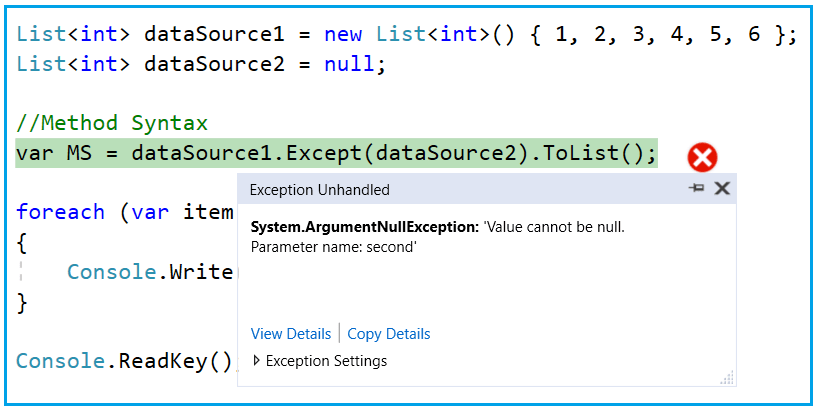##### Example to Understand LINQ Except Method with String Data Type:

In the example below, I show how to use LINQ Except Method with String Data using both Method and Query Syntax. Here, we have a string array of countries, and we need to return the countries from the first collection that are not present in the second collection using Except Method. So, modify the Main Method of the Program class as follows.

```using System;
using System.Linq;
namespace LINQDemo
{
class Program
{
static void Main(string[] args)
{
string[] dataSource1 = { "India", "USA", "UK", "Canada", "Srilanka"};
string[] dataSource2 = { "India", "uk", "Canada", "France", "Japan" };

//Method Syntax
var MS = dataSource1.Except(dataSource2).ToList();

//Query Syntax
var QS = (from country in dataSource1
select country)
.Except(dataSource2).ToList();

foreach (var item in QS)
{
Console.WriteLine(item);
}

}
}
}```

Now run the application, and you should get the following output.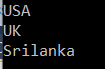Despite having the country UK in the second collection, it still shows in the output. This is because the default comparer used to filter the data by the Except Method is case-insensitive. So, if you want to ignore the case-sensitive, you need to use the other overloaded version of the Except() method, which takes IEqualityComparer as an argument. So, modify the Main Method of the Program as shown below. Here, we are passing StringComparer as an argument, and this StringComparer class already implements the IEqualityComparer interface.

```using System;
using System.Linq;
namespace LINQDemo
{
class Program
{
static void Main(string[] args)
{
string[] dataSource1 = { "India", "USA", "UK", "Canada", "Srilanka"};
string[] dataSource2 = { "India", "uk", "Canada", "France", "Japan" };

//Method Syntax
var MS = dataSource1.Except(dataSource2, StringComparer.OrdinalIgnoreCase).ToList();

//Query Syntax
var QS = (from country in dataSource1
select country)
.Except(dataSource2, StringComparer.OrdinalIgnoreCase).ToList();

foreach (var item in MS)
{
Console.WriteLine(item);
}

}
}
}
```

Now, run the application, and it will display the data as expected.

##### LINQ Except() Method with Complex Type in C#:

The LINQ Except() Method in C# works slightly differently when working with complex types such as Employee, Product, Student, etc. Let us understand this with an example. Create a class file named Student.cs and copy and paste the following code.

```namespace LINQDemo
{
public class Student
{
public int ID { get; set; }
public string Name { get; set; }
}
}
```

This is a very simple student class with just two properties. Let’s say we have the following two data sources.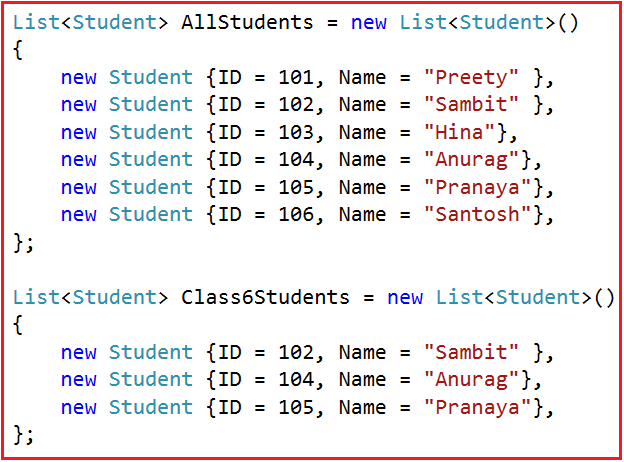As you can see in the above image, we have two data sources. The first data source, i.e., AllStudents, holds the information of all the students, while the second data source, i.e., Class6Students, holds the data of only the 6th-class students. Now, we only need to fetch all the student names except the 6th-class students. That means we need to fetch the student names from the AllStudents data source, which are not present in the second data source, i.e., Class6Students.

```using System.Collections.Generic;
using System;
using System.Linq;
namespace LINQDemo
{
class Program
{
static void Main(string[] args)
{
List<Student> AllStudents = new List<Student>()
{
new Student {ID = 101, Name = "Preety" },
new Student {ID = 102, Name = "Sambit" },
new Student {ID = 103, Name = "Hina"},
new Student {ID = 104, Name = "Anurag"},
new Student {ID = 105, Name = "Pranaya"},
new Student {ID = 106, Name = "Santosh"},
};

List<Student> Class6Students = new List<Student>()
{
new Student {ID = 102, Name = "Sambit" },
new Student {ID = 104, Name = "Anurag"},
new Student {ID = 105, Name = "Pranaya"},
};

//Method Syntax
var MS = AllStudents.Select(x => x.Name).Except(Class6Students.Select(y => y.Name)).ToList();

//Query Syntax
var QS = (from std in AllStudents
select std.Name).Except(Class6Students.Select(y => y.Name)).ToList();

foreach (var name in MS)
{
Console.WriteLine(name);
}

}
}
}```
###### Output: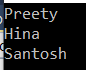Now, we need to select all the students’ information from the first data source, which is not present in the second data source. Let us modify the Main Method of the program class as shown below.

```using System.Collections.Generic;
using System;
using System.Linq;
namespace LINQDemo
{
class Program
{
static void Main(string[] args)
{
List<Student> AllStudents = new List<Student>()
{
new Student {ID = 101, Name = "Preety" },
new Student {ID = 102, Name = "Sambit" },
new Student {ID = 103, Name = "Hina"},
new Student {ID = 104, Name = "Anurag"},
new Student {ID = 105, Name = "Pranaya"},
new Student {ID = 106, Name = "Santosh"},
};

List<Student> Class6Students = new List<Student>()
{
new Student {ID = 102, Name = "Sambit" },
new Student {ID = 104, Name = "Anurag"},
new Student {ID = 105, Name = "Pranaya"},
};

//Method Syntax
var MS = AllStudents.Except(Class6Students).ToList();

//Query Syntax
var QS = (from std in AllStudents
select std).Except(Class6Students).ToList();

foreach (var student in MS)
{
Console.WriteLine(\$" ID : {student.ID} Name : {student.Name}");
}

}
}
}
```
###### Output: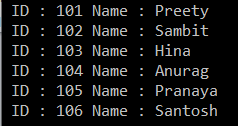As you can see, it displays all the student’s data from the first data source. This is because the default comparer used for comparison only checks whether two object references are equal. It will not check the individual property values of the complex object. In our previous article, we discussed the many ways to solve the above problem. Now, let us see some ways to solve the above problem.

##### Using Anonymous Type with Except Method in C#:

In this approach, we need to select all the individual properties to an anonymous type. The following program does exactly the same thing.

```using System.Collections.Generic;
using System;
using System.Linq;
namespace LINQDemo
{
class Program
{
static void Main(string[] args)
{
List<Student> AllStudents = new List<Student>()
{
new Student {ID = 101, Name = "Preety" },
new Student {ID = 102, Name = "Sambit" },
new Student {ID = 103, Name = "Hina"},
new Student {ID = 104, Name = "Anurag"},
new Student {ID = 105, Name = "Pranaya"},
new Student {ID = 106, Name = "Santosh"},
};

List<Student> Class6Students = new List<Student>()
{
new Student {ID = 102, Name = "Sambit" },
new Student {ID = 104, Name = "Anurag"},
new Student {ID = 105, Name = "Pranaya"},
};

//Method Syntax
var MS = AllStudents.Select(x => new {x.ID, x.Name })
.Except(Class6Students.Select(x => new { x.ID, x.Name })).ToList();

//Query Syntax
var QS = (from std in AllStudents
select new { std.ID, std.Name})
.Except(Class6Students.Select(x => new { x.ID, x.Name })).ToList();

foreach (var student in QS)
{
Console.WriteLine(\$" ID : {student.ID} Name : {student.Name}");
}

}
}
}
```

Now run the application, and it should display the output as expected, as shown in the below image.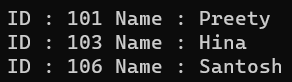Let us see how to achieve the same thing using Comparer.

##### Using IEqualityComparer Comparer with LINQ Except Method in C#:

In this approach, we need to create a class, and then we need to implement the IEqualityComparer interface, and we need to implement the Equals and GetHashCode methods. So, create a class file named StudentComparer.cs and copy and paste the following code.

```using System.Collections.Generic;
using System;
namespace LINQDemo
{
public class StudentComparer : IEqualityComparer<Student>
{
public bool Equals(Student x, Student y)
{
//First check if both object reference are equal then return true
if(object.ReferenceEquals(x, y))
{
return true;
}

//If either one of the object refernce is null, return false
if (object.ReferenceEquals(x,null) || object.ReferenceEquals(y, null))
{
return false;
}

//Comparing all the properties one by one
return x.ID == y.ID && x.Name == y.Name;
}

public int GetHashCode(Student obj)
{
//If obj is null then return 0
if (obj == null)
{
return 0;
}

//Get the ID hash code value
int IDHashCode = obj.ID.GetHashCode();

//Get the Name HashCode Value
int NameHashCode = obj.Name == null ? 0 : obj.Name.GetHashCode();

return IDHashCode ^ NameHashCode;
}
}
}
```

Next, we need to create an instance of the StudentComparer class and pass that instance to the Except method. So, modify the Main method of the Program class as follows.

```using System.Collections.Generic;
using System;
using System.Linq;
namespace LINQDemo
{
class Program
{
static void Main(string[] args)
{
List<Student> AllStudents = new List<Student>()
{
new Student {ID = 101, Name = "Preety" },
new Student {ID = 102, Name = "Sambit" },
new Student {ID = 103, Name = "Hina"},
new Student {ID = 104, Name = "Anurag"},
new Student {ID = 105, Name = "Pranaya"},
new Student {ID = 106, Name = "Santosh"},
};

List<Student> Class6Students = new List<Student>()
{
new Student {ID = 102, Name = "Sambit" },
new Student {ID = 104, Name = "Anurag"},
new Student {ID = 105, Name = "Pranaya"},
};

//Create an instance of StudentComparer
StudentComparer studentComparer = new StudentComparer();

//Method Syntax
var MS = AllStudents
.Except(Class6Students, studentComparer).ToList();

//Query Syntax
var QS = (from std in AllStudents
select std)
.Except(Class6Students, studentComparer).ToList();

foreach (var student in MS)
{
Console.WriteLine(\$" ID : {student.ID} Name : {student.Name}");
}

}
}
}
```

Now run the application, and it should display the output as expected, as shown in the below image.##### Overriding Equals() and GetHashCode() Methods within the Student Class

In C#.NET, any type (predefined or user-defined) is inherited from the Object class by default. That means the Student class is also inherited from the Object class. And we also know that the Object class provides virtual methods such as Equals() and GetHashCode(). So, now, we need to override the Object class’s Equals() and GetHashCode() methods within the Student class. So, modify the Student class as shown below. We are overriding the Equals() and GetHashCode() methods here.

```namespace LINQDemo
{
public class Student
{
public int ID { get; set; }
public string Name { get; set; }

public override bool Equals(object obj)
{
//As the obj parameter type is object, so we need to
//cast it to Student Type
return this.ID == ((Student)obj).ID && this.Name == ((Student)obj).Name;
}

public override int GetHashCode()
{
//Get the ID hash code value
int IDHashCode = this.ID.GetHashCode();
//Get the string HashCode Value
//Check for null refernece exception
int NameHashCode = this.Name == null ? 0 : this.Name.GetHashCode();
return IDHashCode ^ NameHashCode;
}
}
}```

With the above changes in place, now modify the Main method of the Program class as shown below. Now, we need to use the overloaded version of the Except method, which does not take any parameter.

```using System.Collections.Generic;
using System;
using System.Linq;
namespace LINQDemo
{
class Program
{
static void Main(string[] args)
{
List<Student> AllStudents = new List<Student>()
{
new Student {ID = 101, Name = "Preety" },
new Student {ID = 102, Name = "Sambit" },
new Student {ID = 103, Name = "Hina"},
new Student {ID = 104, Name = "Anurag"},
new Student {ID = 105, Name = "Pranaya"},
new Student {ID = 106, Name = "Santosh"},
};

List<Student> Class6Students = new List<Student>()
{
new Student {ID = 102, Name = "Sambit" },
new Student {ID = 104, Name = "Anurag"},
new Student {ID = 105, Name = "Pranaya"},
};

//Method Syntax
var MS = AllStudents
.Except(Class6Students).ToList();

//Query Syntax
var QS = (from std in AllStudents
select std)
.Except(Class6Students).ToList();

foreach (var student in QS)
{
Console.WriteLine(\$" ID : {student.ID} Name : {student.Name}");
}

}
}
}```

Now execute the above program, and it will display the output as expected, as shown in the below image.##### Implementing IEquatble<T> Interface in Student Class.

In this approach, we need to implement the IEquatble<T> Interface in Student Class, and we need to implement the Equals Method of the IEquatble<T> Interface. We also need to override the GetHashCode method of the Object class. So, modify the Student class as shown below.

```using System;
namespace LINQDemo
{
public class Student : IEquatable<Student>
{
public int ID { get; set; }
public string Name { get; set; }

public bool Equals(Student other)
{
return this.ID.Equals(other.ID) && this.Name.Equals(other.Name);
}
public override int GetHashCode()
{
int IDHashCode = this.ID.GetHashCode();
int NameHashCode = this.Name == null ? 0 : this.Name.GetHashCode();
return IDHashCode ^ NameHashCode;
}
}
}```

As you can see, here we have done two things. First, we implement the Equals method of the IEquatable interface, and then we override the GetHashCode method of the Object class. With the above changes in place, now modify the Main Method of the Program class as shown below.

```using System.Collections.Generic;
using System;
using System.Linq;
namespace LINQDemo
{
class Program
{
static void Main(string[] args)
{
List<Student> AllStudents = new List<Student>()
{
new Student {ID = 101, Name = "Preety" },
new Student {ID = 102, Name = "Sambit" },
new Student {ID = 103, Name = "Hina"},
new Student {ID = 104, Name = "Anurag"},
new Student {ID = 105, Name = "Pranaya"},
new Student {ID = 106, Name = "Santosh"},
};

List<Student> Class6Students = new List<Student>()
{
new Student {ID = 102, Name = "Sambit" },
new Student {ID = 104, Name = "Anurag"},
new Student {ID = 105, Name = "Pranaya"},
};

//Method Syntax
var MS = AllStudents
.Except(Class6Students).ToList();

//Query Syntax
var QS = (from std in AllStudents
select std)
.Except(Class6Students).ToList();

foreach (var student in QS)
{
Console.WriteLine(\$" ID : {student.ID} Name : {student.Name}");
}

}
}
}```

Run the application, and you should see the output as expected, as shown in the below image.##### When to Use LINQ Except Method in C#?

Here are some common scenarios where you might want to use the Except method:

• Filtering out Unwanted Elements: If you have a list of items and want to exclude certain elements contained in another list, Except is an efficient way to accomplish that.
• Data Comparison: When comparing data from different sources or time periods, Except can help identify which items have been removed or are no longer present.
• Creating Unique Sets: If you need to ensure that a collection contains unique elements not found in another collection, Except can serve as a way to enforce this.
• Syncing Data: When syncing data between systems, you might use Except to determine which items must be added or deleted to make one system’s dataset match another’s.
• Auditing Changes: If you have two versions of a list (e.g., before and after some operation), Except can help you identify what has changed between the two.

In the next article, I will discuss the LINQ Intersect Method in C# with Examples. In this article, I explain the LINQ Except Method in C# with Examples. I hope you enjoy this article and understand the LINQ Except Method in C# with Examples.

### 2 thoughts on “LINQ Except Method in C#”

1.“If we want to retrieve the elements such as 2, 4, and 6 from the first data source which does not exist in the second data source then we need to apply the distinct operation.”

Did you mean except operation ?

1.Hi,
It’s a Typo Error. We have corrected this. Thanks for identifying the type error.